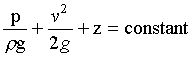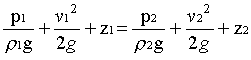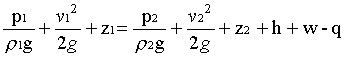### Bernoulli Equation

Home -> Lecture Notes -> Fluid Mechanics -> Unit-II--> 1

This is the basic from of Bernoulli equation for steady incompressible inviscid flows. It may be written for any two points 1 and 2 on the same streamline as--> 2

The contstant of Bernoulli equation, can be named as total head (ho) has different values on different streamlines.--> 3

The total head may be regarded as the sum of the piezometric head h* = p/rg + z and the kinetic head v2/2g.

Bernoullie equation is arrived from the following assumptions:

1. Steady flow - common assumption applicable to many flows.
2. Incompressible flow - acceptable if the flow Mach number is less than 0.3.
3. Frictionless flow - very restrictive; solid walls introduce friction effects.
4. Valid for flow along a single streamline; i.e., different streamlines may have different ho.
5. No shaft work - no pump or turbines on the streamline.
6. No transfer of heat - either added or removed.

Range of validity of the Bernoulli Equation:

Bernoulli equation is valid along any streamline in any steady, inviscid, incompressible flow. There are no restrictions on the shape of the streamline or on the geometry of the overall flow. The equation is valid for flow in one, two or three dimensions.

Modifications on Bernoulli equation:

Bernoulli equation can be corrected and used in the following form for real cases.where 'q' is the work done by pump and 'w' is the work done by the fluid, and h is the head loss by friction.